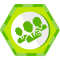JSTL 学习、应用记录 转

JSP 标准标记库（ Standard Tag Library ， JSTL) 是一组以标准化格式实现许多通用的 Web 站点功能的定制标记。

JSP 技术的优势之一在于其定制标记库工具。除了核心 JSP 标记，如 jsp:include 之外，定制标记库工具使您能够创建唯一的标记来描述以站点或应用程序为导向的操作。一旦您创建了定制标记库 ( 简称 taglib) ，当您扩展项目或转向另一个项目时您可以重复使用这些标记。您还可以将 taglib 传递给其它开发人员，从而他们可以在自己的网站上使用，或者在您 Web 应用程序的其它部分使用。

JSTL 的目标是为了简化 JSP 页面的设计。对于页面设计人员来说，使用脚本语言（默认值是 JAVA 语言）操作动态数据是比较困难的，而采用标签和表达式语言相对容易一些， JSTL 的使用为页面设计人员和程序开发人员的分工协作提供了便利。

<%@ taglib prefix="c" uri="http://java.sun.com/jsp/jstl/core" %>

<%@ taglib prefix="sql" uri="http://java.sun.com/jsp/jstl/sql" %>

<%@ taglib prefix="fmt" uri="http://java.sun.com/jsp/jstl/fmt" %>

Core 标签库主要包括了一般用途的标签、条件标签、迭代标签和 URL 相关的标签。在 JSP 页面使用Core 标签，要使用 taglig 指令，指定引用的标签库，如下：

<%@ taglib rui=”http://java.sun.com/jsp/jstl/core”  prefix=”c” %>

1.<c:out>

2.<c:set>

<c:set var=”username” value=”lisi” scope=”session”/>

3.<c:remove>

4.<c:catch>

Eg ：

<c:catch var=”exception”>

<%

int i = 5;

int j = 0;

int k=i/j;

%>

</c:catch>

<c:out value=”\${exception}” /><br>

<c:out value=”\${exception.massage}”/>

1.<c:if>

<c:if test=”\${user.visitCount==1}”>

This is your first visit .

</c:if>

<c:out value=”\${result}” />

2.<c:choose>

<c:choose> 和 <c:when> 、 <c:otherwise> 一起实现互斥条件执行，类似于 java 中的 if else.

<c:choose> 一般作为 <c:when> 、 <c:otherwise> 的父标签。

eg ：

<c:choose>

<c:when test="\${row.v_money<10000}">

初学下海

</c:when>

<c:when test="\${row.v_money>=10000&&row.v_money<20000}">

身手小试

</c:when>

<c:otherwise>

商业能手

</c:otherwise>

</c:choose>

// 遍历记录集

<c:forEach items="\${finalResult.rows}" var="row">

<tr class="<%=tdClass[(rank+1)%2]%>">

<td align="center"><span><%=rank%> </span></td>

<td align="center"><span ><c:out value="\${row.player_name}"/></span> </td>

<td align="center"><span > ￥ <c:out value="\${row.money}"/></span></td>

</tr>

<%rank++;%>

</c:forEach>

<c:forEach var =”i” begin=”100 “ end= “110”>

\${i}

</c:forEach>

// 将数据库某查询的结果声明为一个变量

<sql:query var="finalResult" >

select player_name,money from tb_player order by money DESC LIMIT 10

</sql:query>

然后可以：

<c:forEach items="\${ finalResult.rows}" var="row" varStatus="s">

</c:forEach>

数据更新标签：

<sql:update>

call proc_set_role_salespro(?,?,?,?,?);

<sql:param value="30"/>

<sql:param value="39"/>

<sql:param value="<%=spID%>"/>

<sql:param value="<%=productID%>"/>

<sql:param value="1"/>

</sql:update>

<sql:query var="queryAllChannelCount">

SELECT COUNT(*) as total FROM tb_channel WHERE game_id=? AND begin_round<func_cur_round(?) AND player_id=? AND channel_flag=0

<sql:param value="\${gameID}"/>

<sql:param value="\${gameID}"/>

<sql:param value="\${playerID}"/>

</sql:query>

<c:forEach items="\${queryAllChannelCount.rowsByIndex}" var="channelCN">

<c:set value="\${channelCN}" var="channelTotal"/>

</c:forEach>

<c:if test="\${param.changsubmit!=null}" >

<c:forEach items="\${paramValues.pro_id}" var="getpro_id" varStatus="getparamsta">

<sql:update>

call proc_set_role_product(?,?,?,?,?,?,?,?);

<sql:param value="\${gameID}"/>

<sql:param value="\${playerID}"/>

<sql:param value="\${getpro_id}"/>

<sql:param value="\${getpro_id}"/>

<sql:param value="\${paramValues.pro_sort[getparamsta.index]}"/>

<sql:param value="\${paramValues.price[getparamsta.index]}"/>

<sql:param value="\${paramValues.output[getparamsta.index]}"/>

<sql:param value="0"/>

</sql:update>

</c:forEach>

</c:if>

<fmt: formatNumber value =”12.3” pattern=”.000”/>

<fmt:formatDate value=”<%=new java.util.Date() %>” type=”date” />

格式化的结果是： 2007-5-27 .

<fmt:formatDate value=”<%=new java.util.Date() %>” type=”time” />

<fmt:formatDate value=”<%=new java.util.Date() %>” type=”both” />

1. 替换 request.getParameter("test"):

<c:if test="\${param.test!=null}" >

<c:out value="\${param.test}" />

</c:if>

2. <c:redirect url="a.jsp">

3.<c:redirect url="/max.jsp" context="/ch16">

<c:param name="name1" value="665"/>

<c:param name="name3" value=" 斯蒂芬 "/>

</c:redirect>

4.<c:forTokens items="zhangsan:lisi:as" delims=":" var="name">

\${name}

</c:forTokens>汪纬2011/05/11
31
0
URL访问Java程序-----Servlet

2018/07/16
11
0

dengzhangtao
2010/12/06
286
0
Jsp 和 Servlet 有什么不同？

2018/08/22
155
3
StandardWrapper分析-tomcat6.x源码阅读

2013-10-20 StandardWrapper是什么StandardWrapper是负责对Servlet的封装，在tomcat的结构层次中属于最内层，跟Servlet最接近的组件，是装载Servlet的容器，StandardWrapper没有子容器，因为...

douglaswei
2013/11/19
89
0

Podman 使用指南

> 原文链接：Podman 使用指南 Podman 原来是 CRI-O 项目的一部分，后来被分离成一个单独的项目叫 libpod。Podman 的使用体验和 Docker 类似，不同的是 Podman 没有 daemon。以前使用 Docker...

6
0

Airship

7
0

LNMP环境介绍 Nginx 处理的请求有两种，分为 静态与动态 图片，js，css，视频，音频，flash 等都是静态请求，这些数据都不是保存在数据库里面的 动态请求一般来说，需要的数据是在数据库里面...

doomcat

2
0

popgis

7
0

code-ortaerc

4
0